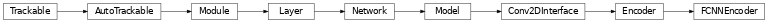FCNNEncoder¶

Inheritance Diagramclass ashpy.models.convolutional.encoders.FCNNEncoder(layer_spec_input_res, layer_spec_target_res, kernel_size, initial_filters, filters_cap, encoding_dimension)[source]

Fully Convolutional Encoder.

Output a 1x1xencoding_size vector. The output neurons are linear.

Examples

• Direct Usage:

dummy_generator = FCNNEncoder(
layer_spec_input_res=(64, 64),
layer_spec_target_res=(8, 8),
kernel_size=5,
initial_filters=4,
filters_cap=128,
encoding_dimension=100,
)
print(dummy_generator(tf.zeros((1, 64, 64, 3))).shape)

(1, 1, 1, 100)

Methods

 __init__(layer_spec_input_res, …) Instantiate the FCNNDecoder.

Attributes

 activity_regularizer Optional regularizer function for the output of this layer. dtype dynamic inbound_nodes Deprecated, do NOT use! Only for compatibility with external Keras. input Retrieves the input tensor(s) of a layer. input_mask Retrieves the input mask tensor(s) of a layer. input_shape Retrieves the input shape(s) of a layer. input_spec Gets the network’s input specs. layers losses Losses which are associated with this Layer. metrics Returns the model’s metrics added using compile, add_metric APIs. metrics_names Returns the model’s display labels for all outputs. name Returns the name of this module as passed or determined in the ctor. name_scope Returns a tf.name_scope instance for this class. non_trainable_variables non_trainable_weights outbound_nodes Deprecated, do NOT use! Only for compatibility with external Keras. output Retrieves the output tensor(s) of a layer. output_mask Retrieves the output mask tensor(s) of a layer. output_shape Retrieves the output shape(s) of a layer. run_eagerly Settable attribute indicating whether the model should run eagerly. sample_weights state_updates Returns the updates from all layers that are stateful. stateful submodules Sequence of all sub-modules. trainable trainable_variables Sequence of variables owned by this module and it’s submodules. trainable_weights updates variables Returns the list of all layer variables/weights. weights Returns the list of all layer variables/weights.
__init__(layer_spec_input_res, layer_spec_target_res, kernel_size, initial_filters, filters_cap, encoding_dimension)[source]

Instantiate the FCNNDecoder.

Parameters: layer_spec_input_res (tuple of (int, int)) – Shape of the input tensors. layer_spec_target_res – (tuple of (int, int)): Shape of tensor desired as output of _get_layer_spec(). kernel_size (int) – Kernel used by the convolution layers. initial_filters (int) – Numbers of filters to used as a base value. filters_cap (int) – Cap filters to a set amount, in the case of an Encoder is a ceil value AKA the max amount of filters. encoding_dimension (int) – encoding dimension. None ValueError – If filters_cap < initial_filters